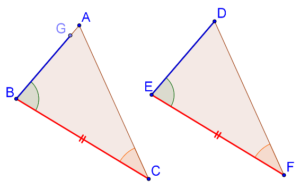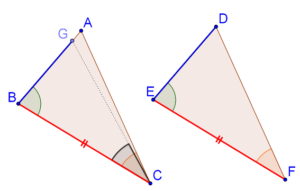# The ASA Criterion Proof

Go back to  'Triangles-Quadrilaterals'

For this purpose, consider triangles ABC and DEF once again, where BC = EF, ∠B = ∠E and ∠C = ∠F. Note that this will also mean that ∠A = ∠D (can you see why?). Now, we have to show that these two triangles are congruent. Here is a step-by-step proof.

Step 1: If AB is equal to DE, then the two triangles would be congruent by the SAS criterion. So, let us suppose that AB is not equal to DE. Then, one of them would be greater than the other – say that AB > DE.

Step 2: Mark a point on AB (call it G), such that GB = DE, as shown below:Step 3: We note that ∆GBC is congruent to ∆DEF by the SAS criterion. This means that ∠BCG = ∠F.Step 4: Finally, we note that ∠F = ∠C (given), and so ∠BCG = ∠C. Is that possible if CG is in a different direction than CA? No, it’s not! This means that CG must be in the same direction as CA, or in other words, G and A coincide, or: GB = AB = DE. Thus, the two triangles are congruent by the SAS criterion.

An important aspect of the proof of the ASA criterion we have encountered is that it builds on the SAS criterion – it assumes the truth of the SAS criterion.